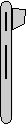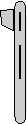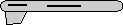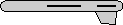# Maths - Axis-Angle - Sample Orientations

In order to try to explain things and give some examples we can try I thought it might help to show the rotations for a finite subset of the rotation group. We will use the set of rotations of a cube onto itself, this is a permutation group which gives 24 possible rotations as explaned on this page.

Heading applied first giving 4 possible orientations:reference orientation angle = 0 degrees axis = 1,0,0rotate by 90 degrees about y axis angle = 90 degrees axis = 0,1,0rotate by 180 degrees about y axis angle = 180 degrees axis = 0,1,0rotate by 270 degrees about y axis angle = 90 degrees axis = 0,-1,0 or angle = -90 degrees axis = 0,1,0

Then apply attitude +90 degrees for each of the above: (note: that if we went on to apply bank to these it would just rotate between these values, the straight up and straight down orientations are known as singularities because they can be fully defined without using the bank value)angle = 90 degrees axis = 0,0,1angle = 120 degrees axis = 0.5774,0.5774,0.5774angle = 180 degrees axis = 0.7071,0.7071,0angle = 120 degrees axis = -0.5774,-0.5774,0.5774

Or instead apply attitude -90 degrees (also a singularity):angle = 90 degrees axis = 0,0,-1 (equivalent rotation to: angle = -90 degrees axis = 0,0,1)angle = 120 degrees axis = -0.5774,0.5774,-0.5774angle = 180 degrees axis = -0.7071,0.7071,0angle = 120 degrees axis = 0.5774,-0.5774,-0.5774

Normally we don't go beyond attitude + or - 90 degrees because these are singularities, instead apply bank +90 degrees:angle = 90 degrees axis = 1,0,0angle = 120 degrees axis = 0.5774,0.5774,-0.5774angle = 180 degrees axis = 0,0.7071,-0.7071angle = 120 degrees axis = 0.5774,-0.5774,0.5774

Apply bank +180 degrees:angle = 180 degrees axis = 1,0,0angle = 180 degrees axis = 0.7071,0,-0.7071angle = 180 degrees axis = 0,0,1angle = 180 degrees axis = 0.7071,0,0.7071

Apply bank -90 degrees:angle = 90 degrees axis = -1,0,0 (equivalent rotation to: angle = -90 degrees axis = 1,0,0)angle = 120 degrees axis = -0.5774,0.5774,0.5774angle = 180 degrees axis = 0,0.7071,0.7071angle = 120 degrees axis = -0.5774,-0.5774,-0.5774

encoding of these rotations in quaternions is shown here.
encoding of these rotations in matrices is shown here.
encoding of these rotations in euler angles is shown here.

 metadata block see also: Correspondence about this page Book Shop - Further reading. Where I can, I have put links to Amazon for books that are relevant to the subject, click on the appropriate country flag to get more details of the book or to buy it from them.3D Math Primer - Aimed at complete beginners to vector and matrix algebra. Other Math Books

This site may have errors. Don't use for critical systems.